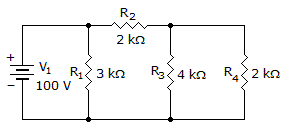# Electronics - Series-Parallel Circuits - Discussion

### Discussion :: Series-Parallel Circuits - True or False (Q.No.2)

2.

The voltage across R3 is 20 volts.[A]. True [B]. False

Explanation:

No answer description available for this question.

 Rahul Vhanmurgi said: (Nov 10, 2014) HOW ITS FALSE?

 Niranjan said: (Feb 6, 2015) We get current in mesh 3 & 2 are 11.11 & 16.66 amps respectively. So difference we get as 5.55 & vol is 4*5.55 which is not 20 so false.

 Jayson said: (May 8, 2018) Complete a Thevinen equivalent of V1 and R1. Giving you a current source of 100/3000 = 33.3mA in series with 3k+2k = 5k . Now do the Norton equivalent circuit, 33.3mA * 5k = 166.7V. Then all 3 resistors are in parallel R12, R3, R4. So the voltage across each is 167V.

 Nairu said: (Sep 20, 2019) Since on R1 is in parallel with the voltage source, its voltage is 100v. This 100 volts will pass to the 2nd resistor. Since the second is in series with the third one. We can use voltage divider theorem. That is VR3=(R3/(R2+R3))*V. Substitue and you'll get 66.67V. It is not equal to 20 volts. So it's false.